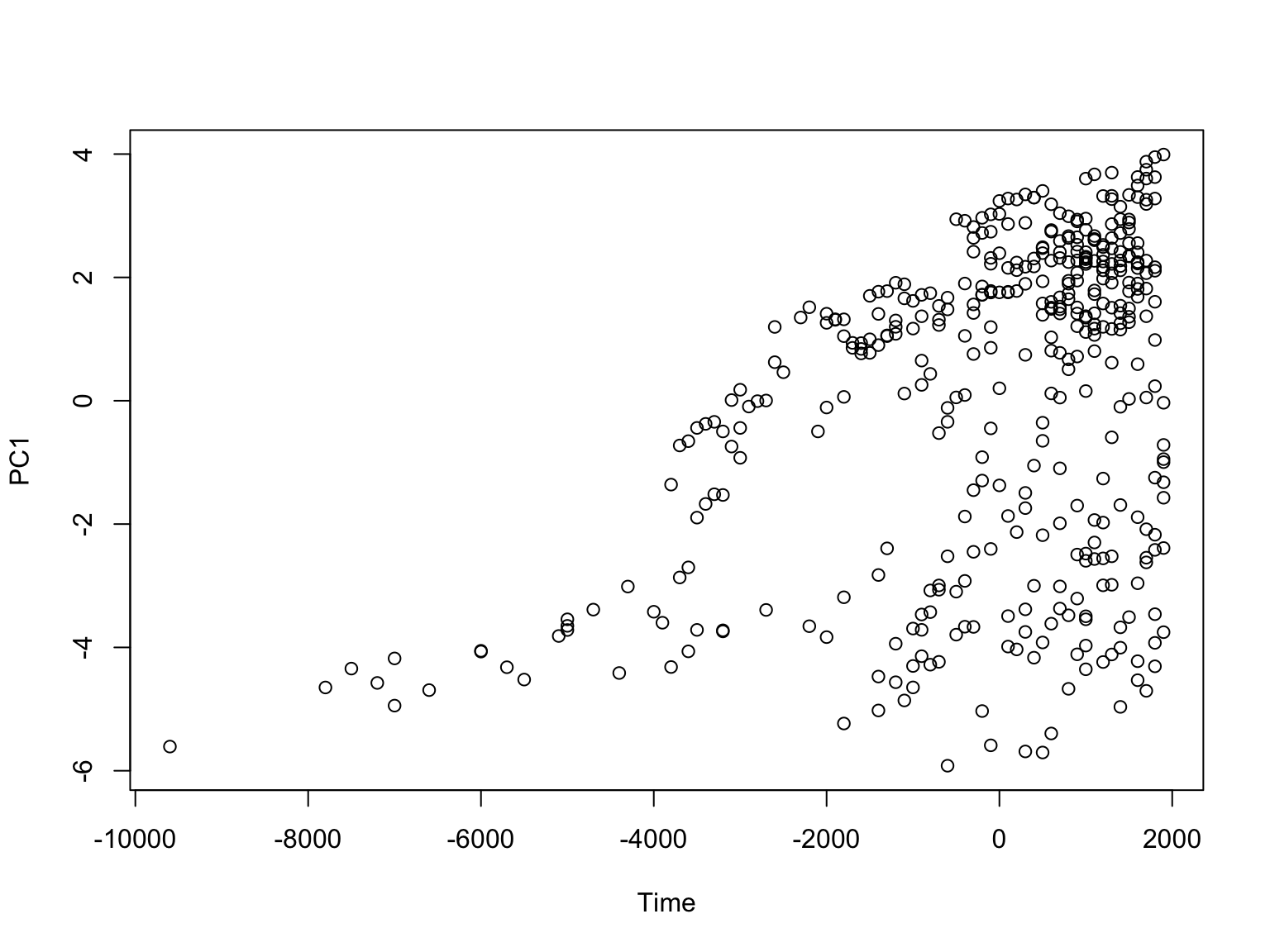# Principal Components Analysis

11 September 2019

$\newcommand{\X}{\mathbf{x}} \newcommand{\w}{\mathbf{w}} \newcommand{\V}{\mathbf{v}} \newcommand{\S}{\mathbf{s}} \newcommand{\Expect}{\mathbb{E}\left[ #1 \right]} \newcommand{\Var}{\mathrm{Var}\left[ #1 \right]} \newcommand{\SampleVar}{\widehat{\mathrm{Var}}\left[ #1 \right]} \newcommand{\Cov}{\mathrm{Cov}\left[ #1 \right]} \DeclareMathOperator{\tr}{tr} \DeclareMathOperator*{\argmin}{argmin}$

# Previously…

• Represent items in our data base as vectors of numerical features
• Want to look for patterns in those features
• First, or as an example, low-dimensional structure in the vectors

# What the data looks like

• Data is $$\mathbf{x} = n\times p$$ matrix
• Could be multivariate data at $$n$$ time points
• Or multivariate data at $$n$$ locations
• Or scalar data at $$n$$ time points and $$p$$ locations
• Write $$\vec{x}_i$$ for row $$i$$ ($$1\times p$$ matrix)
• First, center the data, just to reduce book-keeping
• i.e., subtract the mean of each column
• Optional: scale each column to equal variance

# Finding the “principal” component

• We don’t want to keep track of $$p$$ dimensions
• We want one dimension
• We also don’t want to distort the data too much
• Pick a direction in the $$p$$-dimensional space, and a length-1 vector $$\vec{w}$$
• What’s the best $$\vec{w}$$?

# Projections

• $$\vec{x}_i \cdot \vec{w} =$$ length of $$\vec{x}_i$$’s projection on to the direction of $$\vec{w}$$
• $$(\vec{x}_i \cdot \vec{w})\vec{w} =$$ the actual projected vector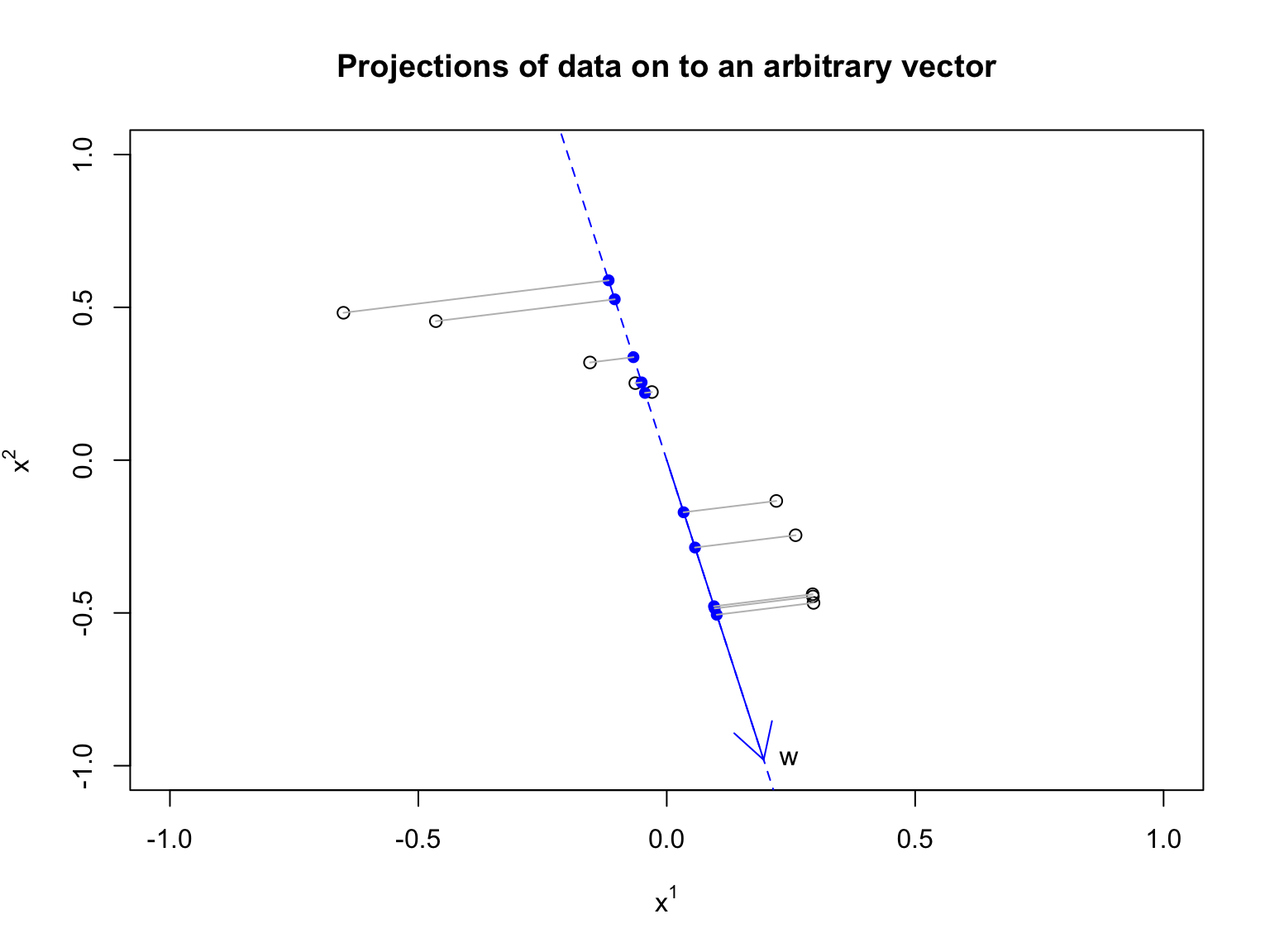# How well does the projection approximate the original?

Do it for one vector first:

$\begin{eqnarray} {\|\vec{x_i} - (\vec{w}\cdot\vec{x_i})\vec{w}\|}^2 & =& \left(\vec{x_i} - (\vec{w}\cdot\vec{x_i})\vec{w}\right)\cdot\left(\vec{x_i} - (\vec{w}\cdot\vec{x_i})\vec{w}\right)\\ & = & \vec{x_i}\cdot\vec{x_i} -\vec{x_i}\cdot (\vec{w}\cdot\vec{x_i})\vec{w}\\ \nonumber & & - (\vec{w}\cdot\vec{x_i})\vec{w}\cdot\vec{x_i} + (\vec{w}\cdot\vec{x_i})\vec{w}\cdot(\vec{w}\cdot\vec{x_i})\vec{w}\\ & = & {\|\vec{x_i}\|}^2 -2(\vec{w}\cdot\vec{x_i})^2 + (\vec{w}\cdot\vec{x_i})^2\vec{w}\cdot\vec{w}\\ & = & \vec{x_i}\cdot\vec{x_i} - (\vec{w}\cdot\vec{x_i})^2 \end{eqnarray}$

# How well does the projection approximate the original?

• Add up across all the data vectors:
$\begin{eqnarray} MSE(\vec{w}) & = & \frac{1}{n}\sum_{i=1}^{n}{\|\vec{x_i}\|^2 -{(\vec{w}\cdot\vec{x_i})}^2}\\ & = & \frac{1}{n}\left(\sum_{i=1}^{n}{\|\vec{x_i}\|^2} -\sum_{i=1}^{n}{(\vec{w}\cdot\vec{x_i})^2}\right) \end{eqnarray}$
• First bit doesn’t depend on $$\vec{w}$$, so doesn’t matter for minimizing
• So we want to maximize $L(\vec{w}) = \frac{1}{n}\sum_{i=1}^{n}{{(\vec{w}\cdot\vec{x_i})}^2}$

# Minimizing MSE is maximizing variance

$\begin{eqnarray} L(w) & = & \frac{1}{n}\sum_{i=1}^{n}{{(\vec{w}\cdot\vec{x_i})}^2}\\ & = & {\left(\frac{1}{n}\sum_{i=1}^{n}{\vec{x_i}\cdot\vec{w}}\right)}^2 + \SampleVar{\vec{w}\cdot\vec{x_i}} \end{eqnarray}$

($$\Expect{Z^2} = (\Expect{Z})^2 + \Var{Z}$$)

But $\frac{1}{n}\sum_{i=1}^{n}{\vec{x_i} \cdot \vec{w}} = 0$ (Why?)

# Minimizing MSE is maximizing variance

so $L(\vec{w}) = \SampleVar{\vec{w}\cdot\vec{x_i}}$

# Minimizing MSE is maximizing variance

The direction which gives us the best approximation of the data is the direction with the greatest variance

# OK, how do we find this magic direction?

Matrix form: all lengths of projections $$=\mathbf{x}\mathbf{w}$$ $$[n\times 1]$$

$\begin{eqnarray} \SampleVar{\vec{w}\cdot\vec{x_i}} & = & \frac{1}{n}\sum_{i}{{\left(\vec{x_i} \cdot \vec{w}\right)}^2}\\ & = & \frac{1}{n}{\left(\X \w\right)}^{T} \left(\X \w\right)\\ & = & \frac{1}{n} \w^T \X^T \X \w\\ & = & \w^T \frac{\X^T \X}{n} \w\\ \end{eqnarray}$

Fact: $$\V \equiv \frac{\X^T \X}{n} =$$ sample covariance matrix of the vectors

# OK, how do we find this magic direction?

• We need to maximize $\begin{equation} \SampleVar{\vec{w}\cdot\vec{x_i}} = \w^T \V \w \end{equation}$
• Constraint: $$\vec{w}$$ has length 1 $$\Leftrightarrow$$ $$\w^T \w = 1$$

• Add a Lagrange multiplier $$\lambda$$ and take derivatives

$\begin{eqnarray} \mathcal{L}(\w,\lambda) & \equiv & \w^T\V\w - \lambda(\w^T \w -1)\\ \frac{\partial \mathcal{L}}{\partial \lambda} & = & \w^T \w -1\\ \frac{\partial \mathcal{L}}{\partial \w} & = & 2\V\w - 2\lambda\w \end{eqnarray}$
• Set derivatives to zero:
$\begin{eqnarray} \w^T \w & = & 1\\ \V \w & = & \lambda \w \end{eqnarray}$

# The magic direction is an eigenvector

$\begin{eqnarray} \w^T \w & = & 1\\ \V \w & = & \lambda \w \end{eqnarray}$

THIS IS AN EIGENVALUE/EIGENVECTOR EQUATION!

At the solution, $\SampleVar{\vec{w}\cdot\vec{x_i}} = \w^T \V \w = \w^T \lambda \w = \lambda$ so the maximum is the leading eigenvector of $$\V$$

# About the sample covariance matrix

$$\V$$ is a special matrix: symmetric and non-negative definite:

• Eigenvalues are all real (b/c symmetric)
• If $$\lambda_i \neq \lambda_j$$, then $$v_i \perp v_j$$ (b/c symmetric)
• Eigenvectors form a basis, and (can be chosen to be) orthonormal
• All $$\lambda_i \geq 0$$ (b/c non-negative definite)
$\begin{eqnarray} \text{Lead eigenvector of} \V & = & 1^{\mathrm{st}} \text{principal component}\\ & = & \text{Direction of maximum variance}\\ & = & \text{Best 1D approximation to the data} \end{eqnarray}$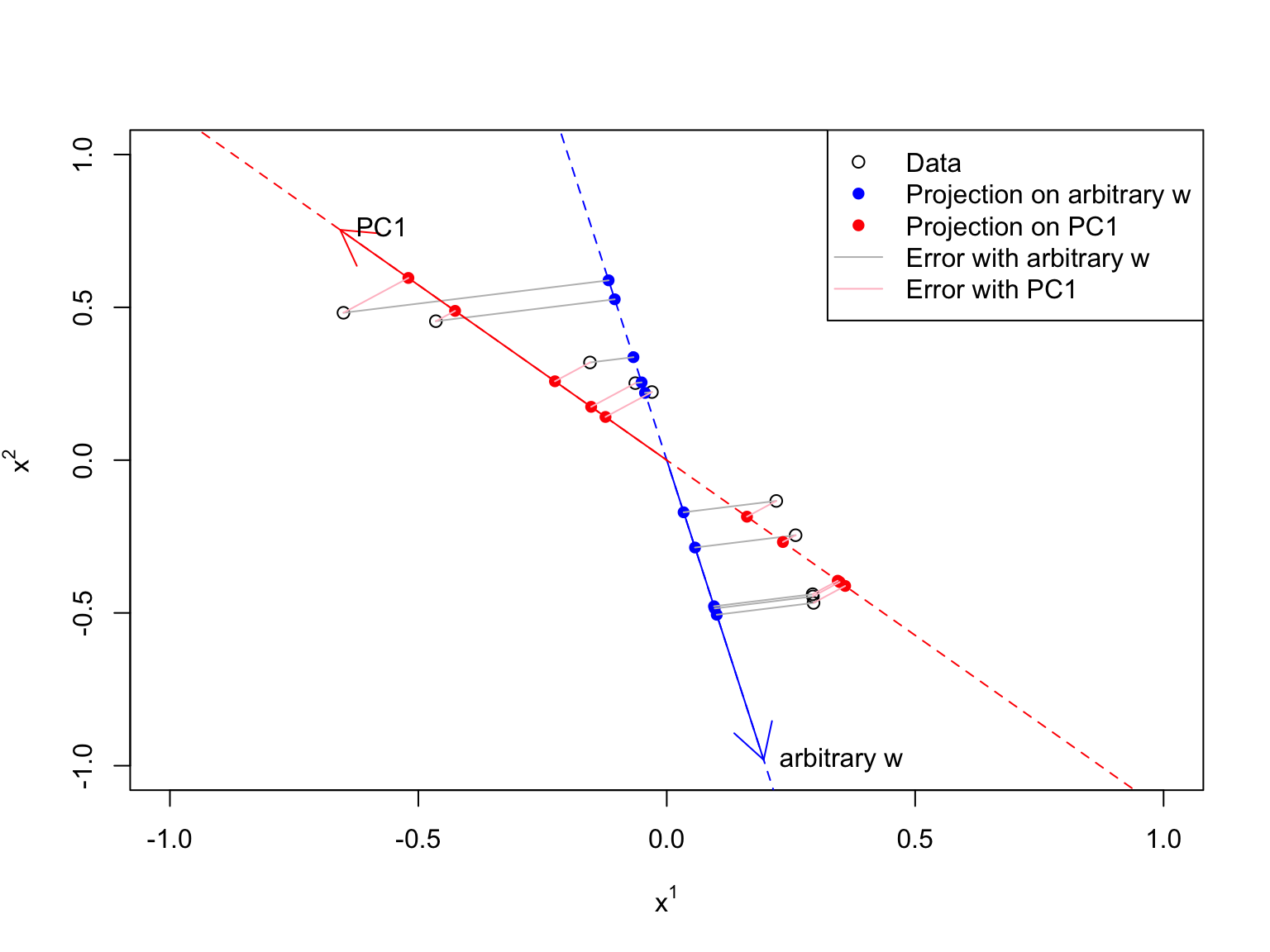# Multiple principle components

• What about approximating by a plane, hyper-plane, hyper-hyper-plane, etc.?
• Intuition: take the direction of maximum variance $$\perp$$ the first principal component
• Then direction of maximum variance $$\perp$$ the first two principal components
• These are the eigenvectors of $$\V$$, in order of decreasing $$\lambda$$
• Gory details go at the end of these slides

# Some properties of the eigenvalues

• All eigenvalues $$\geq 0$$
• In general, $$p$$ non-zero eigenvalues
• If the data are exactly in a $$q$$-dimensional subspace, then exactly $$q$$ non-zero eigenvalues
• If $$n < p$$, at most $$n$$ non-zero eigenvalues
• Two points define a line, three define a plane, …

# Some properties of the PCs

• The principal components are orthonormal
• $$\vec{w}_i \cdot \vec{w}_i = 1$$
• $$\vec{w}_i \cdot \vec{w}_j = 0$$ (unless $$i=j$$)
• $$\w^T\w = \mathbf{I}$$
• PC1 is the direction of maximum variance through the data
• That variance is $$\lambda_1$$, biggest eigenvalue of $$\V$$
• PC $$i+1$$ is the direction of maximum variance $$\perp$$ PC1, PC2, $$\ldots$$ PC $$i$$
• That variance is $$\lambda_{i+1}$$

# Some properties of PC scores

$\S = \X \w$

• Average score on each PC $$=0$$ (b/c we centered the data)
$\begin{eqnarray} \Var{\text{scores}} & = & \frac{1}{n} \S^T \S\\ \end{eqnarray}$

Show that this $$=\mathbf{\Lambda}$$

(Hint: $$\V = \w \mathbf{\Lambda} \w^T$$)

# Some properties of PC scores

$\S = \X \w$

• Average score on each PC $$=0$$ (b/c we centered the data)
$\begin{eqnarray} \Var{\text{scores}} & = & \frac{1}{n} \S^T \S\\ & = & \frac{1}{n} (\X\w)^T(\X\w)\\ & = & \frac{1}{n}\w^T \X^T \X \w\\ & = & \w^T \V\w = \w^T (\w \mathbf{\Lambda} \mathbf{w}^T) \w & = & (\w^T \w) \mathbf{\Lambda} (\w^T\w)\\ & = & \mathbf{\Lambda} \end{eqnarray}$
• Variance of score on PC $$i$$ $$=\lambda_i$$ (by construction)
• Covariance of score on PC $$i$$ with score on PC $$j$$ $$=0$$

# Some properties of PCA as a whole

• If we use all $$p$$ principal components, we have the eigendecomposition of $$\V$$: $\V = \w \mathbf{\Lambda} \mathbf{w}^T$ $$\mathbf{\Lambda}=$$ diagonal matrix of eigenvalues $$\lambda_1, \ldots \lambda_p$$
• If we use all $$p$$ principal components, $\X = \S\w^T$
• If we use only the top $$q$$ PCs, we get:
• the best rank-$$q$$ approximation to $$\V$$
• the best dimension-$$q$$ approximation to $$\X$$

# Another way to think about PCA

• The original coordinates are correlated
• There is always another coordinate system with uncorrelated coordinates
• We’re rotating to that coordinate system
• Rotating to new coordinates $$\Rightarrow$$ multiplying by an orthogonal matrix
• That matrix is $$\mathbf{w}$$
• The new coordinates are the scores

# Interpreting PCA results

• PCs are linear combinations of the original coordinates
• Good idea to scale variables with different units of measurement first
• $$\Rightarrow$$ PCs change as you add or remove coordinates
• Put in 1000 measures of education and PC1 is education…
• Very tempting to reify the PCs
• i.e., to “make them a thing”
• sometimes totally appropriate…
• sometimes not at all appropriate…
• Be very careful when the only evidence is the PCA

# PCA is exploratory analysis, not statistical inference

• We assumed no model
• Pro: That’s the best linear approximation, no matter what
• Con: doesn’t tell us how much our results are driven by noise
• Prediction: PCA predicts nothing
• Inference: If $$\V \rightarrow \Var{X}$$ then PCs $$\rightarrow$$ eigenvectors of $$\Var{X}$$
• But PCA doesn’t need this assumption
• Doesn’t tell us about uncertainty
• Next time, will look at a PCA-like model: factor models

# In R

• prcomp is the best built-in PCA command
• Slightly complicated object returned
• Understand it with a real data example from a recent, high-profile paper

# Example: The axis of historical complexity

Turchin et al. (2018)

9 variable measuring social complexity, over time, for dozens of locations across the world

Dates from -9600 to 1900

summary(soccomp[, complexities], digits = 2)
##      PolPop       PolTerr          CapPop        levels      government
##  Min.   :1.4   Min.   :-0.22   Min.   :1.4   Min.   :0.0   Min.   :0.00
##  1st Qu.:4.2   1st Qu.: 3.65   1st Qu.:3.5   1st Qu.:1.8   1st Qu.:0.24
##  Median :6.0   Median : 5.18   Median :4.3   Median :3.0   Median :0.62
##  Mean   :5.5   Mean   : 4.78   Mean   :4.2   Mean   :2.9   Mean   :0.55
##  3rd Qu.:6.8   3rd Qu.: 5.97   3rd Qu.:5.1   3rd Qu.:4.0   3rd Qu.:0.86
##  Max.   :8.5   Max.   : 7.40   Max.   :6.3   Max.   :6.6   Max.   :1.00
##     infrastr       writing         texts          money
##  Min.   :0.00   Min.   :0.00   Min.   :0.00   Min.   :0.0
##  1st Qu.:0.34   1st Qu.:0.26   1st Qu.:0.10   1st Qu.:1.8
##  Median :0.75   Median :0.82   Median :0.93   Median :4.0
##  Mean   :0.64   Mean   :0.65   Mean   :0.63   Mean   :3.4
##  3rd Qu.:0.90   3rd Qu.:0.86   3rd Qu.:0.97   3rd Qu.:5.0
##  Max.   :1.00   Max.   :1.00   Max.   :1.00   Max.   :6.0

# Example: The axis of historical complexity

soccomp.pca <- prcomp(soccomp[, complexities], scale = TRUE)

What are the parts of the return value?

str(soccomp.pca)
## List of 5
##  $sdev : num [1:9] 2.634 0.733 0.646 0.581 0.481 ... ##$ rotation: num [1:9, 1:9] 0.351 0.32 0.339 0.341 0.332 ...
##   ..- attr(*, "dimnames")=List of 2
##   .. ..$: chr [1:9] "PolPop" "PolTerr" "CapPop" "levels" ... ## .. ..$ : chr [1:9] "PC1" "PC2" "PC3" "PC4" ...
##  $center : Named num [1:9] 5.515 4.779 4.229 2.923 0.552 ... ## ..- attr(*, "names")= chr [1:9] "PolPop" "PolTerr" "CapPop" "levels" ... ##$ scale   : Named num [1:9] 1.59 1.561 1.112 1.449 0.325 ...
##   ..- attr(*, "names")= chr [1:9] "PolPop" "PolTerr" "CapPop" "levels" ...
##  $x : num [1:414, 1:9] -4.35 -4.24 -4.11 -3.67 -3.51 ... ## ..- attr(*, "dimnames")=List of 2 ## .. ..$ : NULL
##   .. ..$: chr [1:9] "PC1" "PC2" "PC3" "PC4" ... ## - attr(*, "class")= chr "prcomp" • sdev = standard deviations along PCs $$=\sqrt{\lambda_i}$$ • rotation = matrix of eigenvectors $$=\w$$ • x = scores on all PCs $$=\S$$ # Example: The axis of historical complexity plot(cumsum(soccomp.pca$sdev^2)/sum(soccomp.pca$sdev^2), xlab = "Number of components used", ylab = expression(R^2), ylim = c(0, 1)) abline(h = 0.75, lty = "dashed") abline(h = 0.9, lty = "dotted")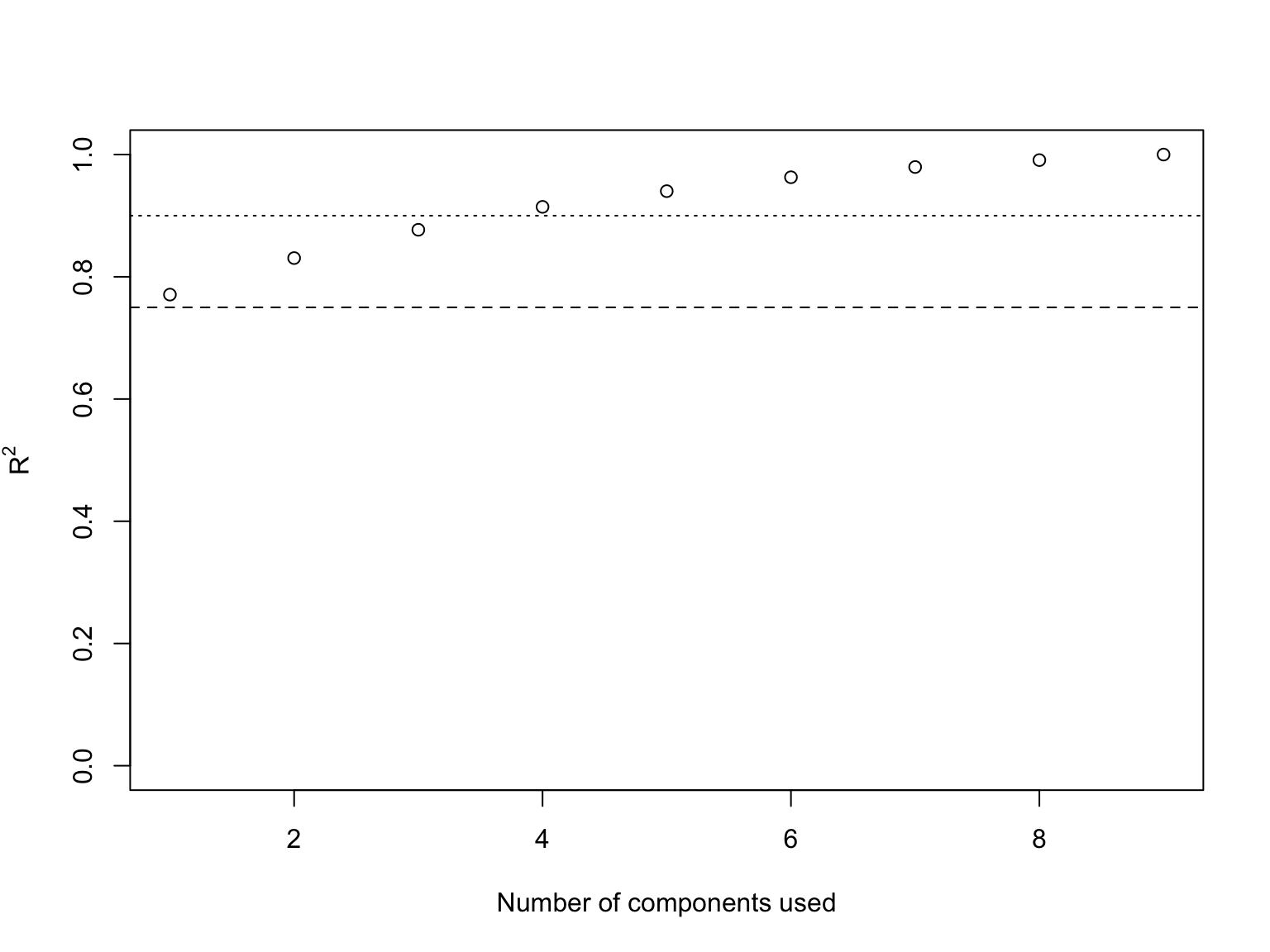One principal component already keeps more than 75% of the variance (3 components keep just under 90%) # Example: The axis of historical complexity plot(soccomp.pca$rotation[, 1], xlab = "", main = "PCs of the complexity measures",
xaxt = "n", ylab = "PC weight", ylim = c(-1, 1), type = "l")
axis(side = 1, at = 1:9, labels = colnames(soccomp)[complexities], las = 2,
cex.axis = 0.5)
lines(soccomp.pca$rotation[, 2], col = "red") lines(soccomp.pca$rotation[, 3], col = "blue")
legend("bottomright", legend = c("PC1", "PC2", "PC3"), lty = "solid", col = c("black",
"red", "blue"))
abline(h = 0, col = "grey")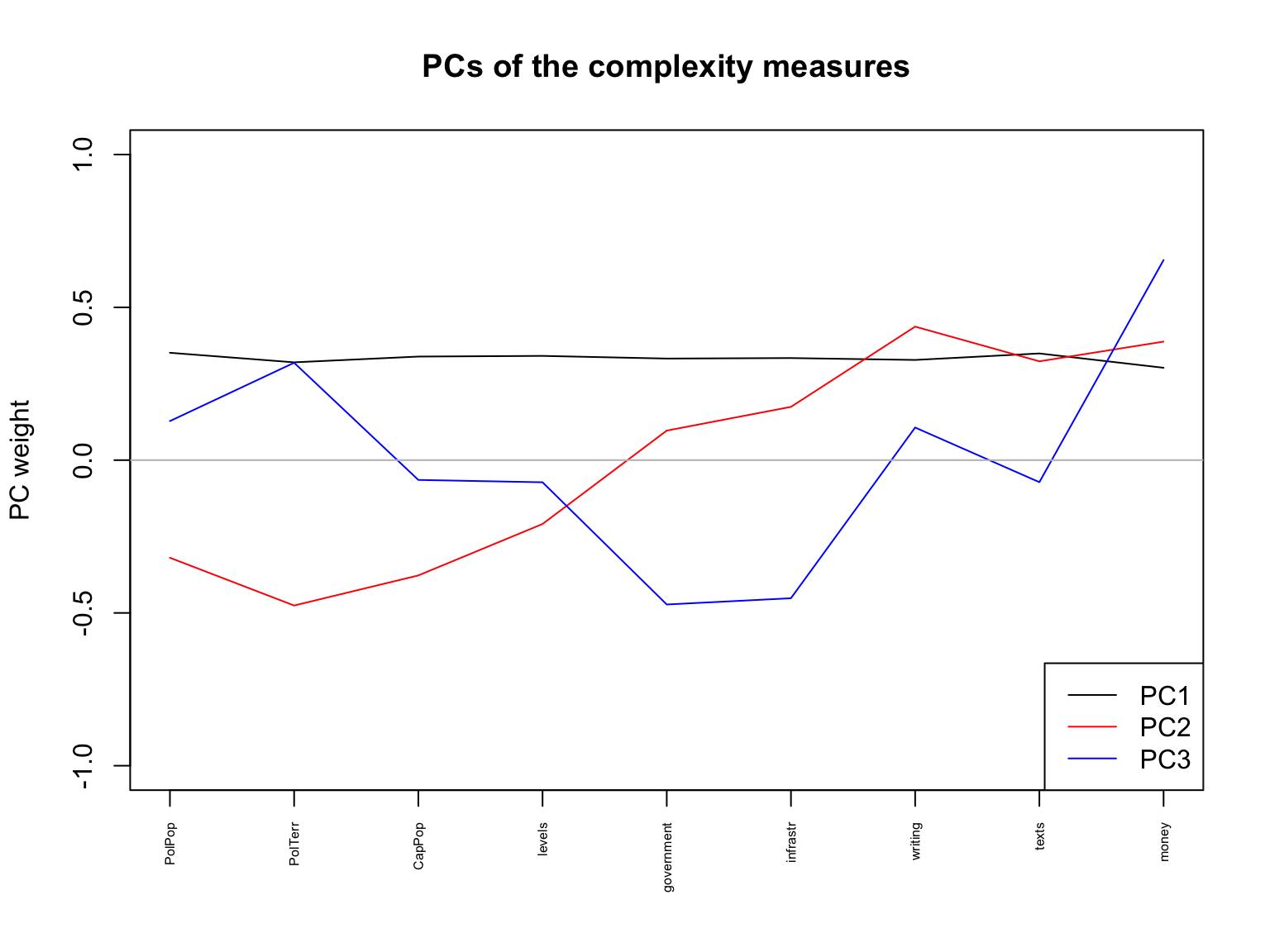• PC1: Nearly equal positive weight on all variables
• High scores: large population, large areas, large capital cities, much hierarchy, very differentiated, etc.
• Low scores: small and unsophisticated
• PC2: negative weight on population, area, levels of hierarchy, positive weight on gov’t, infrastructure, information, money
• High scores: small but sophisticated
• Low scores: big but dumb

# Example: The axis of historical complexity

soccomp$PC1 <- soccomp.pca$x[, 1]
with(soccomp, plot(PC1 ~ Time))